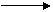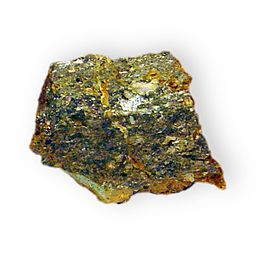10 minutes maximum! Can you do it in 5?

1. ...can only travel a few centimeters in air?

2. ...is only stopped by thick lead?

3. ..has a negative charge?

4. ..has a mass of 4 units and a charge of +2?

5. ..has no charge and no mass?

6. ...can pass through paper?

7. In this decay equation, what are the missing numbers x and y?

 14 C14 N + y ß- 6 x -1
• A. x=5, y=1
• B. x=5, y=0
• C. x=7, y=1
• D. x=7, y=0

8. When Uranium decays it creates Thorium (Th) and emits an alpha particle(α).

Which one of the following equations correctly shows the balanced equation?A. 235 U231 Th + 4 α 92 90 2
 B. 235 U233 Th + 2 α 92 88 4
 C. 235 U231 Th + 4 α 92 92 2
 D. 235 U231 Th + 2 α 92 90 4

9. Which of these is the correct notation for gamma radiation?

 A. 0 γ 1
 B. 0 γ -1
 C. 0 γ 0
 D. 1 γ 0

10. An oxygen isotope emits a ß- particle. This means ..

• A. ..the mass (nucleon) number will decrease by 1, the atomic number will increase by 1.
• B. ..the mass (nucleon) number will decrease by 1, the atomic number will stay the same.
• C. ..the mass (nucleon) number will stay the same, the atomic number will increase by 1.
• D. ..the mass (nucleon) number will stay the same, the atomic number will decrease by 1.